# Force Couples, Pure Moments, Couples and Torques Civil Engineering (CE) Notes | EduRev

## Civil Engineering (CE) : Force Couples, Pure Moments, Couples and Torques Civil Engineering (CE) Notes | EduRev

The document Force Couples, Pure Moments, Couples and Torques Civil Engineering (CE) Notes | EduRev is a part of the Civil Engineering (CE) Course Introduction to Dynamics and Vibrations- Notes, Videos, MCQs.
All you need of Civil Engineering (CE) at this link: Civil Engineering (CE)

2.3 Force Couples, Pure Moments, Couples and Torques

We have seen that a force acting on a rigid body has two effects: (i) it tends to move the body; and (ii) it tends to rotate the body.

A natural question arises – is there a way to rotate a body without moving it? And is there a kind of force that causes only rotation without translation?

The answer to both questions is yes.

2.3.1 Force couples

A system of forces that exerts a resultant moment, but no resultant force, is called a force couple.

The simplest example of a force couple consists of two equal and opposite forces +F and −F acting some distance apart. Suppose that the force −F acts at position r while the force +F acts at position r+ The resultant moment is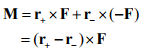Of course, the vector r+ - r- is just the vector from the point where −F acts to the point where +F acts. This gives a quick way to calculate the moment induced by a force couple: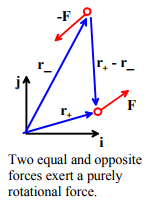The moment induced by two equal and opposite forces is equal to the moment of one force about the point of action of the other. It doesn’t matter which force you use to do this calculation.

Note that a force couple
(i) Has zero resultant force
(ii) Exerts the same resultant moment about all points.

Its effect is to induce rotation without translation.

The effect of a force couple can therefore be characterized by a single vector moment M. The physical significance of M is equivalent to the physical significance of the moment of a force about some point. The direction of M specifies the axis associated with the rotational force. The magnitude of M specifies the intensity of the rotational force.

There are many practical examples of force systems that are best thought of as force-couple systems. They include

1. The forces exerted by your hand on a screw-driver
2. The forces exerted by the tip of a screw-driver on the head of a screw
3. The forces exerted by one part of a constant velocity joint on another
4. Drag forces acting on a spinning propeller

2.3.2 Pure moments, couples and torques.- Definition, Physical Interpretation, and Examples

A pure moment is a rotational force. Its effect is to induce rotation, without translation – just like a force couple.

Couples and torques are other names for a pure moment.

A pure moment is a vector quantity – it has magnitude and direction. The physical significance of the magnitude and direction of a pure moment are completely equivalent to the moment associated with a force couple system. The direction of a moment indicates the axis associated with its rotational force (following the right hand screw convention); the magnitude represents the intensity of the force. A moment is often denoted by the symbols shown in the figure.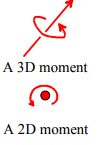The concept of a pure moment takes some getting used to. Its physical effect can be visualized by thinking about our beambalancing problem again.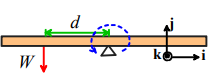The picture above shows the un-balanced beam. We saw earlier that we can balance the beam again by adding a second force, which induces a moment equal and opposite to that of the force W.

We can also balance the beam by applying a pure moment to it. Since the moment of W is dWk , a moment M = −dWk applied anywhere on the beam would balance it.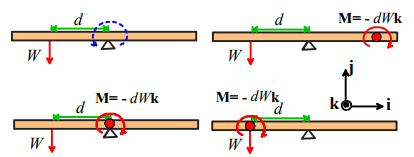You could even apply the moment to the left of the beam – even right on top of the force W if you like!

2.3.3 Units and typical magnitudes of moments

In the SI system, moments have units of Nm (Newton-meters).
In the US system, moments have units of ft-lb (foot pounds)
The conversion factor is 1 Nm = 0.738 ft lb; or 1 ft-lb = 1.356 Nm.
Typical magnitudes are:

• Max torque exerted by a small Lego motor: 0.1 Nm
• Typical torque output of a typical car engine 300-600 Nm
• Breaking torque of a human femur: 140Nm

2.3.4 Measuring Moments

Just as you can buy a force transducer to measure forces, you can buy a force transducer that measures moments. We showed an example of a forcetransducer attached to the wheel of a car during our earlier discussion of force transducers.

Another common moment-measuring system is a torque-wrench. (So then is Oprah a talk wench?) When you tighten the bolts on a precision machine, it’s important to torque them correctly. If you apply too much torque, you will strip the thread. If you don’t apply enough, the bolt will work itself loose during service.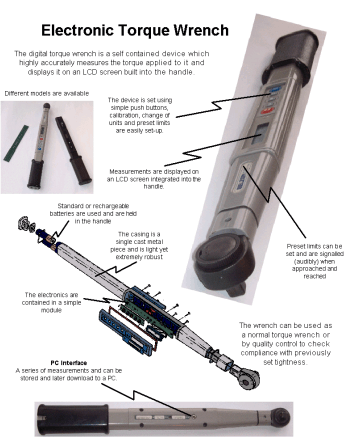You can buy a tool that measures the moment that you apply to a bolt while tightening it. The device may be mechanical, or electronic.

2.3.5 Engineering systems that exert torques

There are many practical examples of moments, or torques, in engineering systems. For example,

(i) The driving axle on your car turns the wheels by exerting a moment on them.
(i) The drive shaft of any motor exerts a torque on whatever it’s connected to. In fact, motors are usually rated by their torque capacity.
(ii) The purpose of a gearbox is to amplify or attenuate torque. You apply a torque to the input shaft, and get a bigger or smaller torque from the output shaft. To do this, the input and output shafts have to rotate at different speeds. There are also some clever gearboxes that allow you to add torques together – they are used in split-power variable speed transmissions, for example.
(iii) A torque converter serves a similar purpose to a gearbox. Unlike a gearbox, however, the input and output shafts don’t rotate at the same speed. The output shaft can be stationary, exerting a large torque, while the input shaft rotates quickly under a modest torque. It is used as part of an automatic transmission system in a car.
(iv) Moments also appear as reaction forces. For example, the resistance you feel to turning the steering wheel of your car is caused by moments acting on the wheels where they touch the ground. The rolling resistance you feel when you ride your bike over soft ground or grass is also due to a moment acting where the wheel touches the ground.
(v) Moments appear as internal forces in structural members or components. For example, a beam will bend because of an internal moment whose direction is transverse to the direction of the beam. A shaft will twist because of an internal moment whose direction is parallel to the shaft. Just as an internal force causes points in a solid to move relative to each other, an internal moment causes points to rotate relative to each other.

Offer running on EduRev: Apply code STAYHOME200 to get INR 200 off on our premium plan EduRev Infinity!

## Introduction to Dynamics and Vibrations- Notes, Videos, MCQs

20 videos|53 docs

,

,

,

,

,

,

,

,

,

,

,

,

,

,

,

,

,

,

,

,

,

,

,

,

,

,

,

;